Students can download 11th Economics Chapter 3 Production Analysis Questions and Answers, Notes, Samcheer Kalvi 11th Economics Guide Pdf helps you to revise the complete Tamilnadu State Board New Syllabus, helps students complete homework assignments and to score high marks in board exams.

## Tamilnadu Samacheer Kalvi 11th Economics Solutions Chapter 3 Production Analysis

### Samacheer Kalvi 11th Economics Production Analysis Text Book Back Questions and Answers

Part – A

Multiple Choice Questions:

Question 1.
The primary factors of production are ………………………..
(a) Labour and Organisation
(b) Labour and Capital
(c) Land and Capital
(d) Land and Labour
(d) Land and LabourQuestion 2.
The man-made physical goods used to produce other goods and services are referred to as.
(a) Land
(b) Labour
(c) Capital
(d) Organization.
(c) Capital

Question 3.
The formula for calculating AP is ………………………
(a) ∆TP/N
(b) ∆TP/∆N
(c) TP/MP
(d) TP/N
(d) TP/NQuestion 4.
Which factor is called the changing agent of the Society
(a) Labourer
(b) Land
(c) Organizer
(d) Capital
(c) Organizer

Question 5.
Who said, that one of the keys of an entrepreneur is “uncertainty – bearing”?
(a) JB Clark
(b) Schumpeter
(c) Knight
(c) KnightQuestion 6.
The functional relationship between “inputs” and “outputs” is called as
(a) Consumption Function
(b) Production Function
(c) Savings Function
(d) Investment Function
(b) Production Function

Question 7.
In a firm 5 units of factors produce 24 units of the product. When the number of factor increases by one, the production increases to 30 units. Calculate the Average Product.
(a) 30
(b) 6
(c) 5
(d) 24
(c) 5

Question 8.
The short-run production is studied through
(a) The Laws of Returns to Scale
(b) The Law of Variable Proportions
(c) Iso-quants
(d) Law of Demand
(b) The Law of Variable ProportionsQuestion 9.
The long-run production function is explained by ………………………
(a) Law of Demand
(b) Law of Supply
(c) Returns to Scale
(d) Law of Variable Proportions
(c) Returns to Scale

Question 10.
An Iso-quant curve is also known as
(a) Inelastic Supply Curve
(b) Inelastic Demand Curve
(c) Equi-marginal Utility
(d) Equal Product Curve
(d) Equal Product Curve

Question 11.
Mention the economies reaped from inside the firm.
(a) Financial
(b) Technical
(c) Managerial
(d) All of the above
(d) All of the aboveQuestion 12.
Cobb-Douglas production function assumes
(a) Increasing returns to scale
(b) Diminishing returns to scale
(c) Constant returns to scale
(d) All of the above
(c) Constant returns to scale

Question 13.
Name the returns to scale when the output increases by more than 5%, for a 5% increase in the inputs.
(a) Increasing returns to scale
(b) Decreasing returns to scale
(c) Constant returns to scale
(d) All of the above
(a) Increasing returns to scaleQuestion 14.
Which of the following is not a characteristic of land?
(a) It’s a limited supply
(b) It is mobile
(c) Heterogeneous
(b) It is mobile

Question 15.
The product obtained from additional factors of production is termed as ……………………….
(a) Marginal product
(b) Total product
(c) Average product
(d) Annual product
(a) Marginal product

Question 16.
Modern economists have propounded the law of
(a) Increasing returns
(b) Decreasing returns
(c) Constant returns
(d) Variable proportions.
(a) Increasing returnsQuestion 17.
Producer’s equilibrium is achieved at the point where ……………………….
(a) Marginal rate of technical substitution (MRTS) is greater the price ratio
(b) MRTS is lesser than the price ratio
(c) MRTS and price ratio are equal to each other
(d) The slopes of isoquant and isocost lines are different.
(c) MRTS and price ratio are equal to each other

Question 18.
The relationship between the price of a commodity and the supply of a commodity is
(a) Negative
(b) Positive
(c) Zero
(d) Increase
(b) PositiveQuestion 19.
If the average product is decreasing, then marginal product ……………………….
(a) Must be greater the average product
(b) Must be less than the average product
(c) Must be increasing
(d) Both a and c
(b) Must be less than average product

Question 20.
A production function measures the relation between
(a) Input prices and output prices
(b) Input prices and the quantity of output
(c) The number of inputs and the quantity of output.
(d) The number of inputs and input prices.
(c) The number of inputs and the quantity of output.

Part – B

Answer the following questions in one or two sentences.

Question 21.
Classify the factors of production?
Factors of production are classified as land, labor, capital, and organization.

1. Land and Labour – Primary factors
2. Capital and organization – Secondary factors.Question 22.
Define Labour.
According to Marshall, labour represents services provided by the factor labour, which helps in yielding an income to the owner of the labor-power.

Question 23.
State the production function?
The production function is the relationship between inputs of productive services and outputs of product per unit of time.Question 24.
Define the Marginal Product of a factor.
Marginal product is the addition made to the total product when one more unit of the variable input is employed.
MP = TP (n)-TP (n- 1)
(OR)
Marginal product is the ratio of the change in the total product to the change in the units of input.
MP = ∆TP / ∆N

Question 25.
What is the Iso – cost line?
An Iso-cost line represents different combinations of inputs which show the same amount of cost.Question 26.
What are the conditions for the producer’s equilibrium?
The Conditions For Producer’S Equilibrium:

1. The Iso-cost line must be tangent to the Iso-quant curve.
2. At the point of tangency, the Iso-quant curve must be convex to the origin or MRTSLK must be declining.

Question 27.
What are the reasons for the upward-sloping supply curve?
As the price of the commodity increases the quantum supplied also increases. So the supply curve has a positive slope.
The quantum supplied of commodity x is represented on X-axis. And the price of the commodity is represented on the Y-axis. The points such as a, b, c, d, and e on the supply curve SS’, represent various quantities at different prices.

1.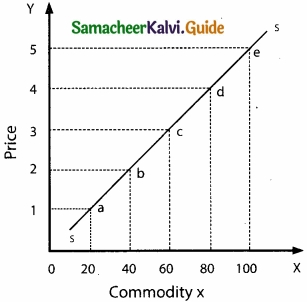PART – C

Answer the following questions in one paragraph.

Question 28.
What are the characteristics of land?
The Characteristics of Land:

1. Land is a primary factor of production.
2. Land is a passive factor of production.
3. Land is the free gift of nature.
4. Land has no cost of production.
5. Land is inelastic in supply.
6. Land is permanent and immovable.
7. Land is heterogeneous and has alternative uses.
8. Land is subject to law of diminishing returns.Question 29.
What are the factors governing the elasticity of supply?
1. Nature of the commodity: The elasticity of supply of durable goods is high but perishables have a low elasticity of supply.

2. Cost of production: Under constant or increasing returns the elasticity of supply is greater, under diminishing returns elasticity is less.

3. Technical condition: In large-scale production, supply cannot be adjusted easily. So elasticity of supply is lesser and vice versa.

4. Time factor: During a very short period, supply cannot be adjusted. In a short period, variable factors can be changed so elasticity is more and in a long period, supply is highly elastic.Question 30.
What are the functions of entrepreneurs?

1. An organizer is the initiator of the business.
2. A successful entrepreneur is always an innovator.
3. An organizer co-ordinates the factors of production to start and run the business or production.
4. An organizer controls and directs the factors to get better results and he supervises for the efficient functioning of all.
5. There are risk-taking and uncertainty bearing obstacles.Question 31.
State and explain the elasticity of supply?
The elasticity of supply may be defined as the degree of responsiveness of change in supply to change in price on the part of sellers.
It is Mathematically expressed as,
Elasticity of supply = Proportionate change in supply / Proportionate change in price
es = $$\frac { \Delta Q_{ S } }{ Q_{ S } }$$ / $$\frac { \Delta P }{ P }$$
es = $$\frac { \Delta Q_{ S } }{ \Delta P }$$ × $$\frac{P}{Qs}$$
Where Qs represents the supply, P represents price, ∆denotes a change.Question 32.
Bring out the Relationship among Total, Average and Marginal Products?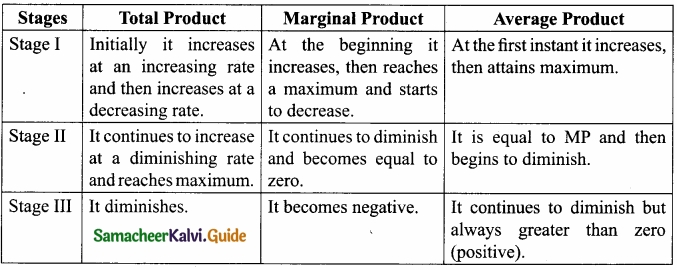Question 33.
Illustrate the concept of producer’s Equilibrium?
Producer equilibrium implies the situation where the producer maximizes his output. It is also known as the optimum combination of the factors of production. In short, the producer manufactures a given amount of output with the ‘least-cost combination of factors’, with his given budget.

Optimum Combination of Factors implies either there is output maximization for given inputs or there is cost minimization for the given output.

Conditions for Producer Equilibrium
The two conditions that are to be fulfilled for the attainment of producer equilibrium are:

1. The isocost line must be tangent to the iso-quant curve.
2. At the point of tangency, the iso-quant curve must be convex to the origin or MRTSLk must be declining.Question 34.
State the Cobb-Douglas Production Function.
The Cobb-Douglas production function was developed by Charles W.Cobb and Paul H. Douglas.
Cobb-Douglas production function describes how much output can be made with capital and labour inputs.
Q = ALα Kβα
Q – output, A – positive constant, K – capital, L – Labour
α and β – Elasticity coefficients of outputs for the inputs,
α + β = 1 denotes constant returns to scale.

PART – D

Question 35.
Examine the Law of Variable Proportions with the help of a diagram?
The law states that if all other factors are fixed and one input is varied in the short run, the total output will increase at an increasing rate at first instance, be constant at a point and then eventually decrease. The marginal product will become negative at last.

According to G.Stigler, “As equal increments of one input are added, the inputs of other productive services being held constant, beyond a certain point, the resulting increments of the product will decrease, i.e., the marginal product will diminish”.

Assumptions:
The Law of Variable Proportions is based on the following assumptions.

1. Only one factor is variable while others are held constant.
2. All units of the variable factor are homogeneous.
3. The product is measured in physical units.
4. There is no change in the state of technology.
5. There is no change in the price of the product.

Total Product (TP):
Total product refers to the total amount of commodity produced by the combination of all inputs in a given period of time. Summation of marginal products, i.e. TP = LMP where, TP = Total Product, MP = Marginal Product

Average Product (TP):
Average Product is the result of the total product divided by the total units of the input employed. In other words, it refers to the output per unit of the input. Mathematically, AP = TP/N Where, AP = Average Product TP = Total Product N = Total units of inputs employed Marginal Product (MP)

Marginal Product is the addition or the increment made to the total product when one more unit of the variable input is employed. In other words, it is the ratio of the change in the total product to the change in the units of the input. It is expressed as MP = ∆TP/∆N

where MP = Marginal Product
TP(n) = Change in total product
∆N = Change in units of input

It is also expressed as
MP = TP (n) – TP (n – 1)
Where MP = Marginal Product
TP(n – 1) = Total product of employing nA unit of a factor
TP(n – 1) = Total product of employing the previous unit of a factor, that is, (n – 1)th unit of a factor.
The Law of Variable Proportions is explained with the help of the following schedule and diagram: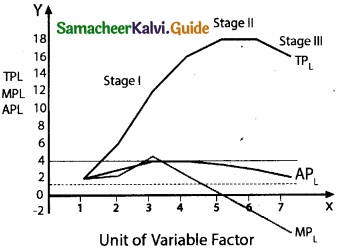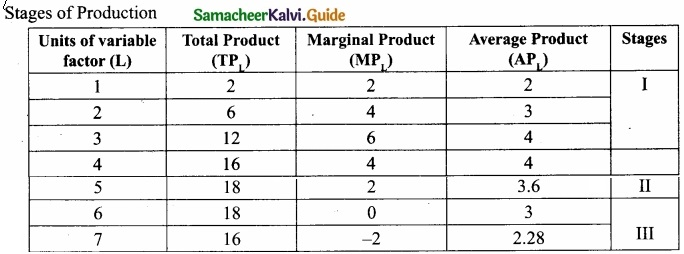In the above table, units of variable factor (labour) are employed along with other fixed factors of production. The table illustrates that there are three stages of production. Though total product increases steadily at the first instant, constant at the maximum point, and then diminishes, it is always positive forever.

While total product increases, the marginal product increases up to a point and then decreases. The total product increases up to the point where the marginal product is zero. When total product tends to diminish marginal product becomes negative.

In the diagram, the number of workers is measured on X-axis while TPL, APL, and MPL are denoted on Y-axis. The diagram explains the three stages of production as given in the above table.

Stage – I
In the first stage, MPL increases up to the third labourer and it is higher than the average product so the total product is increasing at an increasing rate. The tendency of total production to increase at an increasing rate stops at point A and it begins to increase at a decreasing rate. This point is known as ‘Point of Inflexion’.

Stage – II
In the second stage, MPL decreases up to the sixth unit of labor where the MPL curve intersects the X-axis. At the fourth unit of labor MPL = APL. After this, the MPL curve is lower than the APL. TPL increases at a decreasing rate.

Stage – III
The third stage of production shows that the sixth unit of labour is marked by negative MPL, the APL continues to fall but remains positive. After the sixth unit, TPL declines with the employment of more units of the variable factor, labour.Question 36.
List out the properties of isoquants with the help of diagrams?
Properties of Iso – quant Curve: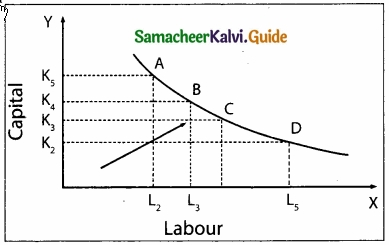1. The isoquant curve has negative slope:
It slopes downwards from left to right indicating that the factors are substitutable. If more of one factor is used, less of the other factor is needed for producing the same level of output.

In the diagram combination, A refers to more capital K5 and less labour L2 As the producer moves to B, C, and D, more labour and less capital are used.

2. Convex to the origin.
This explains the concept of Diminishing Marginal Rate of Technical Substitution (MRTSLK). For example, the capital substituted by 1 unit of labour goes on decreasing when moved from top to bottom. If so, it is called diminishing MRTS. Constant MRTS (straight line) and increasing MRTS (concave) are also possible. It depends on the nature of the isoquant curve.

This means that factors of production are substitutable to each other. The capital substituted per unit of labour goes on decreasing when the isoquant is convex to the origin.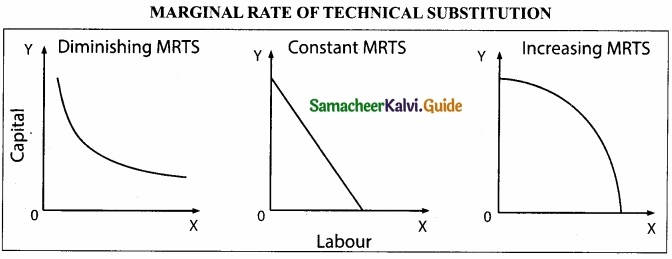3. Nonintersection of Iso – quant curves:
For instance, point A lies on the isoquants IQ1 and IQ2. But the point C shows a higher output and the point B shows a lower level of output IQ1
If C = A, B = A, then C = B. But C > B which is illogical.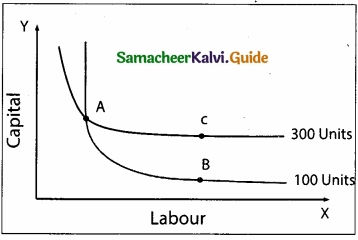4. An upper isoquant curve represents a higher level of output:
Higher IQS show higher outputs and lower IQS show lower outputs, for the upper isoquant curve implies the use of more factors than the lower isoquant curve.
The arrow in the figure shows an increase in the output with a right and upward shift of an isoquant curve.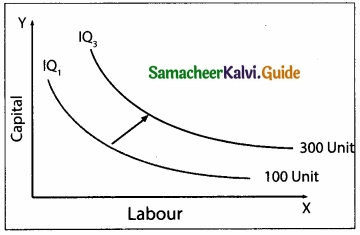5. Isoquant curve does not touch either X-axis or Y-axis:
No isoquant curve touches the X-axis or Y-axis because in IQ1 only capital is used, and in IQ2 only labour is used.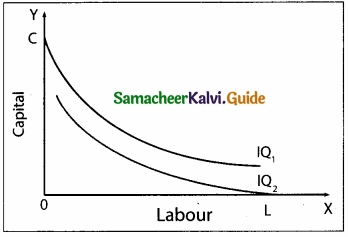Question 37.
Elucidate the Laws of Returns to scale. Illustrate?

In the long-run all factors are variable. The laws of returns to scale explain the relationship between output and the scale of inputs in the long-run when all the inputs are increased in the same proportion.
Assumptions :

1. All the factors are variable except the organization.
2. There is no change in technology.
3. There is perfect competition in the market.
4. Outputs or returns are increased in physical quantities.

Three phases of returns to scale:
1. Increasing returns to scale:
If all inputs are increased by one percent, output increase by more than one percent.

2. Constant returns to scale:
In this case, if all inputs are increased by one percent, output increases by one percent.
Diagrammatic Illustration: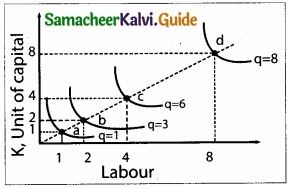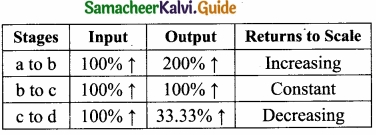The three laws of returns to scale can be explained with the help of the diagram below.

In the figure, the movement from point a to point b represents increasing returns to scale. Because, between these two points output has doubled, but output has tripled. The law of constant returns to scale is implied by the movement from point b to point c.

Because between these two points inputs have doubled and output also has doubled. Decreasing returns to scale are denoted by the movement from the point c to point d since doubling the factors from 4 units to 8 units product less than the increase in inputs, that is by 33.33%.Question 38.
Explain the internal and external economies of scale?
Internal Economies of Scale:

1. Internal Economies of scale refer to the advantages enjoyed by the production unit which causes a reduction in the cost of production of the commodity.
2. For example, a firm enjoying the advantage of an application of most modem machinery, generation of internal capital, and improvement in managerial skill, etc. are sure to reduce the cost of production. They are of various types:

1. Technical Economies:

• When the size of the firm is large, a large amount of capital can be used.
• There is a possibility to introduce up-to-date technologies; this improves the productivity of the firm.
• Research and development strategies can be applied easily.

2. Financial Economies:

• Big firms can float shares in the market for capital expansion, while small firms cannot easily float shares in the market.

3. Managerial Economies:

• Large scale production facilitates specialization and delegation.

4. Labour Economies:

• Large scale production implies greater and minute division of labour.
• This leads to specialization which enhances the quality.
• This increases the productivity of the firm.

5. Marketing Economies:

• In the context of large-scale production, the producers can both buy raw-materials in bulk at a cheaper cost and can take the products to distant markets.
• They enjoy a huge bargaining power.

6. Economies of survival:

• Product diversification is possible when there is large scale production.
• This reduces the risk in production.
• Even if the market for one product collapses, the market for other commodities offsets it.

External Economies of Scale:

1. External Economies of Scale refer to changes in any factor outside the firm causing an improvement in the production process.
2. This can take place in the case of the industry also.
3. These are the advantages enjoyed by all the firms in the industry due to the structural growth.
4. Important external economies of scale are listed below:
• Increased transport facilities
• Banking facilities
• Development of townships
• Development of information and communication.

### Samacheer Kalvi 11th Economics Production Analysis Additional Important Questions and Answers

Part – A

Multiple Choice Questions:

Question 1.
The labour exercised without expecting income is _______
(a) Service
(b) Physical labour
(c) Mental labour
(d) None of the above
(a) ServiceQuestion 2.
Land and Labour are called ……………………. factors.
(a) Primary
(b) Secondary
(c) Territory
(d) Service
(a) Primary

Question 3.
Investment in an advertisement, expenses on capital. training programme are examples of _______ capital
(a) Tangible
(b) Intangible
(c) Visible
(d) Financial
(c) Visible

Question 4.
Reward Paid to capital is ……………………….
(a) Interest
(b) Profit
(c) Wages
(d) Rent
(a) InterestQuestion 5.
Marginal product is
(a) MP = ∆TP / ∆N
(b) MP = ∆AP / ∆N
(c) MP = TP / N
(d) MP = ∆P / N
(a) MP = ∆TP / ∆N

Question 6.
What does a successful entrepreneur will always be made?
(a) Organization
(b) Investment
(c) Capital
(d) Innovation
(d) Innovation

Question 7.
_______ is the exertion of body or mind in the production process.
(a) Labour
(b) Capital
(c) Land
(d) Financial capital
(a) LabourQuestion 8.
Gifts of Nature is called ………………………
(a) Land
(b) Labour
(c) Capital
(d) Production
(a) Land

Question 9.
_______ is the gift of nature.
(a) Labour
(b) Capital
(c) Land
(d) Organization
(c) LandQuestion 10.
Labour cannot be separated from ……………………….
(a) Capital
(b) Labourer
(c) Profit
(d) Organisation
(b) Labourer

Part – B

Answer the following questions in one or two sentences.

Question 1.
What is production?
Production is the process of using various factors of production (inputs)to make output for consumption.Question 2.
Classify the factors determining supply?

1. Price of the commodity
2. Price of other commodities
3. Price of factors
4. Price expectations
5. Technology
6. Natural factors
7. Discovery of new raw materials
8. Taxes and subsidies
9. The objective of the firm

Question 3.
What is capital?
Capital is a produced means of production. – Bohm – Bawerk

Part – C

Answer the following questions in one paragraph.

Question 1.
Explain the difference between internal and external economies?

 Internal Economies of Scale External Economies of Scale 1. Expansion of the firm itself. Expansion of the industry. 2. Lower long-run average cost. Benefits most all firms. 3. Efficiencies from larger-scale production. Agglomeration economies are important. 4. Range of economies (e.g) Technical and Financial. Helps to explain the rapid growth of many cities.Question 2.
What is financial capital?
Financial capital means the assets needed by a firm to provide goods and services measured in terms of monetary value. It is normally raised through debt and equity issues. The prime aim of it is to mass wealth in terms of profit.Question 3.
What are the Supply Function and its assumptions?
The supply of a commodity depends on factors such as the price of the commodity, price of labour, price of capital, the state of technology, number of firms, prices of related goods, and future price expectations, and so on. Mathematically the supply function is QS = f(Px, Pr, Pf, T, O, E)

Where QS = Quantity supplied of x commodity
Px = Price of x Commodity
Pr = Price of related goods
Pf = Price of factors of production
T = Technology
O = Objective of the producer
E = Expected Price of the commodity.

Assumptions:
Law of Supply is based on the following assumptions.

1. There is no change in the prices of factors of production
2. There is no change in the price of capital goods
3. Natural resources and their availability remain the same
4. Prices of substitutes are constant
5. There is no change in technology
6. Climate remains unchanged
7. Political situations remain unchanged
8. There is no change in tax policy

Part – D

Question 1.
What are the diseconomies of scale? Mention its types.
The diseconomies of the scale are a disadvantage to a firm or an industry or an organization. It increases the cost of production. These diseconomies are of two types.

1. Internal diseconomies:
When the scale of production increases beyond the optimum limit, its efficiency may come down.

2. External diseconomies:
It refers to the threat or disturbance to a firm or an industry from factor lying outside it.
For example, a bus strike prevents the easy and correct entry of the workers into the firm. Similarly, the rent of a firm increases very much if new economic units are established in the locality.Question 2.
What are the types of elasticity of supply?
There are five types of elasticity of supply

1. Relatively Elastic Supply:
The coefficient of elastic supply is greater than 1 [ES >1]. One percent change in the price of a commodity causes more than one percent change in the quantity supplied of the commodity.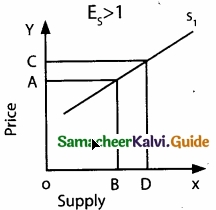2. Unitary Elastic Supply.
The coefficient of elastic supply is equal to 1 [ES = 1]. One percent change in the price of a commodity causes an equal [one percent ] change in the quantity supplied of the commodity.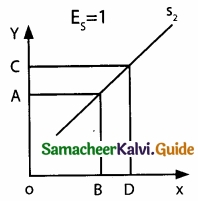3. Relatively Inelastic Supply:
The coefficient of elasticity is less than one [ES < 1], One percent change in the price of a commodity causes a less than one percent change in the quantity supplied of the commodity.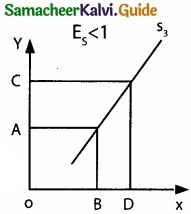4. Perfectly Inelastic Supply:
The coefficient of elasticity is equal to zero [ES = 0], One percent change in the price of a commodity causes no change in the quantity supplied of the commodity.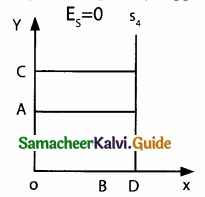5. Perfectly Elastic Supply:
The coefficient of elasticity of supply is infinity [ES – α]. One percent change in the price of a commodity causes an infinite change in the quantity supplied of the commodity.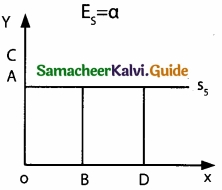ACTIVITY

Question 1.
Visit a market and write a report on the factors that influence the quantity of supply of a commodity of your locality?
Things that cause changes in supply are also called influences of supply.
The influences on supply are:

1. Inputs
2. Productivity
3. Technology
4. Taxes
5. Subsidises
6. Government regulation
7. Number of sellers
8. Political conflict

Supply and Demand Balance:

1. It supply is more price goes down. Demand is more price goes up.
2. Commodity movement get these number [PIS]

P = Production
I = Import
S = Stock

These three represent supply of any commodity = [E × C]
E = Export;
C = Consumption

1. These two represent demand.
2. PIS is more than E × C., then that commodity price goes down.
3. E × C is more than PIS that commodity price goes up.
4. Prediction of any commodity price, you will predict it before that supply-demand imbalance.
5. That means today’s wheat or rice prices are reflections of what will happen to its supply-demand in the next few months rather than its current balance.
6. India’s largest commodity exchange currently working as a trader in Agricultural commodities.
7. The supply is interfered with by legal or illegal cartels.
8. The price is also inflated by opportunistic government charges.
9. A price ceiling prevents a price from rising above the ceiling.
10. Wheat has a price ceiling of 2400 per metric tonne, \$400 is the highest amount supplier can charge.Question 2.
Visit a factory and show how the four factors of production are effectively employed to produce the product in your locality?

1. A Factory is considered capital in the factor of production.
2. Capital is any good that was used to create other goods.
3. A natural resource can’t be land, since it’s not the actual workers within the factory it can’t labor, so it has to be capital.

Factors affecting the location of Industries are as follows:

1. Raw Materials
2. Capital
3. Transport
4. Market
5. Water
6. Power
7. Land
8. Labour
9. Communication

The main factors affecting the location of Industries are as follows:

1. Land
2. Labour
3. Capital
4. Entrepreneurship
• Factors of production refer to the inputs of the production process.
• Factors of production are resources that produce goods and services.

These are four categories of factors of production:

Land:
Natural resources that we use to produce goods and services.

Example:
Oil, gas and goal, water, etc.

Labour:
The work time and work effort that people devote to producing goods and services. This includes human capital which is the quality of the labour forming from knowledge and skills of the person obtained from education, on-the-job training, and work experience.

Capital:

1. The tools, instruments, machine, buildings, and other items that are used to produce goods and services.
2. This includes machinery, hammers, etc.

Entrepreneurship:
The human resource that organizes labour, land, and capital. This includes the idea, plan, etc. about how and what to produce.